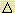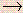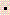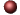ThermodynamicsExamples ofMultiple Choice Questions

1.
Which one of the following thermodynamic quantities is not a state function?
(a) Gibbs free energy
(b) enthalpy
(c) entropy
(d) internal energy
(e) work

2.
At a constant temperature, an ideal gas is compressed from 6.0 liters to 4.0 liters by a constant external pressure of 5.0 atm. How much work is done on the gas?
(a) w = +10 liter atm
(b) w = -10 liter atm
(c) w = +30 liter atm
(d) w = -30 liter atm
(e) The answer cannot be calculated.

3.
A system suffers an increase in internal energy of 80 J and at the same time has 50 J of work done on it. What is the heat change of the system?
(a) +130 J
(b) +30 J
(c) -130 J
(d) -30 J
(e) 0 J

4.
A 5.000 g sample of methanol, CH3OH, was combusted in the presence of excess oxygen in a bomb calorimeter conaining 4000 g of water. The temperature of the water increased from 24.000 oC to 29.765 oC. The heat capacity of the calorimeter was 2657 J/oC. The specific heat of water is 4.184 J/goC. CalculateE for the reaction in kJ/mol.
(a) -314 kJ/mol
(b) -789 kJ/mol
(c) -716 kJ/mol
(d) -121 kJ/mol
(e) -69.5 kJ/mol

5.
A coffee cup calorimeter having a heat capacity of 451 J/oC was used to measure the heat evolved when 0.0300 mol of NaOH(s) was added to 1000 mL of 0.0300 M HNO3 initially at 23.000 oC. The temperature of the water rose to 23.639 oC. CalculateH (in kJ/mol NaNO3) for this reaction. Assume the specific heat of the final solution is 4.18 J/goC; the density of each solution is 1.00 g/mL; and the addition of solid does not appreciably affect the volume of the solution.
HNO3(aq) + NaOH(s)NaNO3(aq) + H2O(l)
(a) -63.7 kJ/mol
(b) -151 kJ/mol
(c) -2.55 kJ/mol
(d) -81.4 kJ/mol
(e) -98.6 kJ/mol

6.
TheHo for the following reaction at 298 K is -36.4 kJ.
1/2 H2(g) + 1/2 Br2(l)HBr(g)
CalculateEo at 298 K. The universal gas constant, R, is 8.314 J/mol K.
(a) -35.2 kJ
(b) +35.2 kJ
(c) -36.4 kJ
(d) -37.6 kJ
(e) +37.6 kJ

7.
Calculate the amount of work done for the conversion of 1.00 mole of Ni to Ni(CO)4 in the reaction below, at 75oC. Assume that the gases are ideal. The value of R is 8.31 J/molK.
Ni(s) + 4 CO (g)Ni(CO)4(g)
(a) 1.80 x 103 J
(b) 8.68 x 103 J
(c) -1.80 x 103 J
(d) -8.68 x 103 J
(e) -494 J

8.
All of the following have a standard heat of formation value of zero at 25oC and 1.0 atm except:
(a) N2(g)
(b) Fe(s)
(c) Ne(g)
(d) H(g)
(e) Hg(l)

9.
For which of the following reactions would theHo for the reaction be labeledHfo?
(a) Al(s) + 3/2 H2(g) + 3/2 O2(g)Al(OH)3(s)
(b) PCl3(g) + 1/2 O2(g)POCl3(g)
(c) 1/2 N2O(g) + 1/4 O2(g)NO(g)
(d) CaO(s) + SO2(g)CaSO3(s)
(e) TheHo for all these reactions would be labeledHfo.

10.
CalculateHo for the reaction:
Na2O(s) + SO3(g)Na2SO4(g)
given the following information:Ho (1) Na(s) + H2O(l)NaOH(s) + 1/2 H2(g) -146 kJ (2) Na2SO4(s) + H2O(l)2NaOH(s) + SO3(g) +418 kJ (3) 2Na2O(s) + 2H2(g)4Na(s) + 2H2O(l) +259 kJ
(a) +255 kJ
(b) -435 kJ
(c) -581 kJ
(d) +531 kJ
(e) -452 kJ

11.
CalculateHorxn for the following reaction at 25.0 oC:
 Fe3O4(s) + CO(g)3FeO(s) + CO2(g)Hfo (kJ/mol) -1118 -110.5 -272 -393.5
(a) -263 kJ
(b) 54 kJ
(c) 19 kJ
(d) -50 kJ
(e) 109 kJ

12.
Calculate the standard heat of formation,Hfo, for FeS2(s), given the following information:
2FeS2(s) + 5O2(g)2FeO(s) + 4SO2(g)Horxn = -1370 kJHfo for SO2(g) = -297 kJ/molHfo for FeO(s) = -268 kJ/mol

(a) -177 kJ
(b) -1550 kJ
(c) -774 kJ
(d) -686 kJ
(e) +808 kJ

13.
Estimate the heat of reaction at 298 K for the reaction shown, given the average bond energies below.
Br2(g) + 3F2(g)2BrF3(g)
 Bond Bond Energy Br-Br 192 kJ F-F 158 kJ Br-F 197 kJ
(a) -516 kJ
(b) -410 kJ
(c) -611 kJ
(d) -665 kJ
(e) -720 kJ

14.
What is the standard entropy change of the reaction below at 298 K with each compound at the standard pressure?
 N2(g) + 3H2(g)2NH3(g) So298 (J/mol K) 191.5 130.6 192.3

(a) -198.7 J/K
(b) 76.32 J/K
(c) 303.2 J/K
(d) -129.7 J/K
(e) 384.7 J/K

15.
The entropy will usually increase when
I. a molecule is broken into two or more smaller molecules.
II. a reaction occurs that results in an increase in the number of moles of gas.
III. a solid changes to a liquid.
IV. a liquid changes to a gas.

(a) I only
(b) II only
(c) III only
(d) IV only
(e) I, II, III, and IV

16.
CalculateGo for the reaction given the following information:
2SO2(g) + O2(g)2SO3(g)Gfo for SO2(g) = -300.4 kJ/molGfo for SO3(g) = -370.4 kJ/mol

(a) -70.0 kJ
(b) +70.0 kJ
(c) -670.8 kJ
(d) -140.0 kJ
(e) +140.0 kJ

17.
For the following reaction at 25oC,Ho = +115 kJ andSo = +125 J/K. CalculateGo for the reaction at 25o.
SBr4(g)S(g) + 2Br2(l)
(a) +152 kJ
(b) -56.7 kJ
(c) +77.8 kJ
(d) +37.1 kJ
(e) -86.2 kJ

18.
The heat of vaporization of freon, CCl2F2, is 17.2 kJ/mol at 25oC. What is the change of entropy for one mole of liquid freon when it vaporizes at 25oC? (Hint: The vaporization process is at equilibrium and what is true forG at equilibrium?)
(a) 57.7 J/K
(b) 0.688 J/K
(c) 5.13 x 103 kJ/K
(d) 3.16 J/K
(e) 239 J/K

19.
Estimate the boiling point of Br2(l) (H = 30.9 kJ;S = 93.0 J/K).
Br2(l)Br2(g)
(a) 85oC
(b) 373oC
(c) 177oC
(d) 59oC
(e) 44oC

20.
For the reaction, A + BC,Ho = +30 kJ;So = +50 J/K.
Therefore the reaction is:
(a) spontaneous at all temperatures.
(b) nonspontaneous at all temperatures.
(c) spontaneous at temperatures less than 600 K.
(d) spontaneous at temperatures greater than 600 K.
(e) spontaneous only at 25oC.

21.
How much heat is absorbed in the complete reaction of 3.00 grams of SiO2 with excess carbon in the reaction below?Ho for the reaction is +624.7 kJ.
SiO2(s) + 3C(s)SiC(s) + 2CO(g)
(a) 366 kJ
(b) 1.13 x 105 kJ
(c) 5.06 kJ
(d) 1.33 x 104 kJ
(e) 31.2 kJ

22.
The standard heat of combustion of ethanol, C2H5OH, is 1372 kJ/mol ethanol. How much heat (in kJ) would be liberated by completely burning a 20.0 g sample?
(a) 686 kJ
(b) 519 kJ
(c) 715 kJ
(d) 597 kJ
(e) 469 kJ

23.
Which statement is incorrect?
(a) At constant pressure,H =E + PV
(b) The thermodynamic symbol for entropy is S.
(c) Gibbs free energy is a state function.
(d) For an endothermic process,H is negative.
(e) If the work done by the system is greater than the heat absorbed by the system,E is negative.

24.
Which statement is false?
(a) The thermodynamic quantity most easily measured in a "coffee cup" calorimeter isH.
(b) No work is done in a reaction occurring in a bomb calorimeter.
(c)H is sometimes exactly equal toE.
(d)H is often nearly equal toE.
(e)H is equal toE for the reaction:
2H2(g) + O2(g)2H2O(g)

1. (e) 2. (a) 3. (b) 4. (c) 5. (e) 6. (d) 7. (b) 8. (d) 9. (a) 10. (c) 11. (c) 12. (a) 13. (a) 14. (a) 15. (e) 16. (d) 17. (c) 18. (a) 19. (d) 20. (d) 21. (e) 22. (d) 23. (d) 24. (e)Click here to return to the top.Choose your next chapter:
Fundamentals of Chemistry | Chemical Formulas & Composition Stoichiometry | Chemical Equations & Rxn Stoichiometry | Types of Chemical Reactions |
| Atomic Structure | Chemical Periodicity | Chemical Bonding | Molecular Structure/Covalent Bonding Theories| Molecular Orbital Theory |
| Acids/Bases/Salts - Theory & Rxns | Acids/Bases/Salts - Calculations (including balancing redox rxns) | Gases | Solids & Liquids | Solutions |
| Thermodynamics | Kinetics | Equilibrium | Aqueous Equilibrium - Acids/Bases/Salts | Aqueous Equilibrium - Buffers & Titrations |
| Aqueous Equilibrium - Slightly Soluble Salts | Electrochemistry | Metallurgy | Metal Properties & Rxns | Nonmetals & Metalloids |
| Coordination Compounds | Nuclear Chemistry | Organic Chem - Formulas/Names/Properties | Organic Chem - Shapes/Rxns/Biopolymers |To report any corrections, please e-mail Dr. Wendy Keeney-Kennicutt.